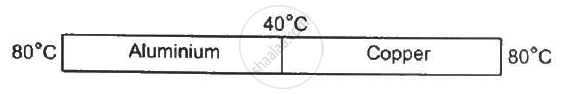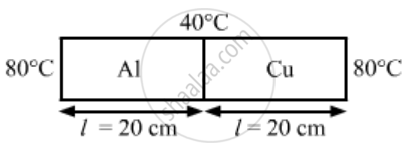Department of Pre-University Education, KarnatakaPUC Karnataka Science Class 11
Advertisement Remove all ads

# Following Figure Shows an Aluminium Rod Joined to a Copper Rod. Each of the Rods Has a Length of 20 Cm and Area of Cross Section 0.20 Cm2. the Junction is Maintained at a Constant Temperature - Physics

Sum

Following Figure shows an aluminium rod joined to a copper rod. Each of the rods has a length of 20 cm and area of cross section 0.20 cm2. The junction is maintained at a constant temperature 40°C and the two ends are maintained at 80°C. Calculate the amount of heat taken out from the cold junction in one minute after the steady state is reached. The conductivites are KAt = 200 W m−1°C−1 and KCu = 400 W m−1°C−1.Advertisement Remove all ads

#### SolutionArea of cross section, A = 0.20 cm2 = 0.2 × 10–4 m2

Thermal conductivity of aluminiumKAl = 200 W/​m ​°C

Thermal conductivity of copper, KCu = 400 W/m​°C

Total heat flowing per second = qAl + qCu

= (K_{AI}xxAxx(80xx40))/l +(K_{cu}xxAxx(80xx40))/l

=(200xx0.2xx10^-4xx40)/0.2 + (400xx0.2xx 10^-40)/0.2

= 8 xx 10^_1 + 16xx10^_1

=24xx10^_1

=2.4  J/s

Heat drawn in 1 minute = 2.4 × 60 = 144 J

Concept: Thermal Expansion of Solids
Is there an error in this question or solution?
Advertisement Remove all ads

#### APPEARS IN

HC Verma Class 11, Class 12 Concepts of Physics Vol. 2
Chapter 6 Heat Transfer
Q 25 | Page 100
Advertisement Remove all ads
Advertisement Remove all ads
Share
Notifications

View all notifications

Forgot password?